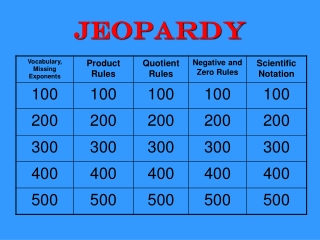DownloadDownload PresentationJeopardy

# Jeopardy

Télécharger la présentation## Jeopardy

- - - - - - - - - - - - - - - - - - - - - - - - - - - E N D - - - - - - - - - - - - - - - - - - - - - - - - - - -
##### Presentation Transcript

1. Jeopardy

2. 100 Write the correct vocabulary term to describe the -5 in (-5x3)

3. 200 Write the correct vocabulary term to describe the x in (-5x3)

4. 300 Write the correct vocabulary term to describe the 3 in (-5x3)

5. 400 Find the missing exponent. (5d4)? = 625d16

6. 500 Find the missing exponent. 5a6 · (10a5)? = 5000a21

7. 100 Simplify. Do not leave any negative exponents in your final answer. x4 · x · x7 · x3 · x9

8. 200 Simplify. Do not leave any negative exponents in your final answer. (–4c7)3

9. 300 Simplify. Do not leave any negative exponents in your final answer. (6x3)(–4x5)

10. 400 Simplify. Do not leave any negative exponents in your final answer. (–7) · (–7)3 · (–7)4

11. 500 Simplify. Do not leave any negative exponents in your final answer. p7x + y · py -2x

12. 100 Simplify. Do not leave any negative exponents in your final answer. 6x4 8x2

13. 200 Simplify. Do not leave any negative exponents in your final answer. 84 · 88 82

14. 300 Simplify. Do not leave any negative exponents in your final answer.

15. 400 Simplify. Do not leave any negative exponents in your final answer.

16. 500 Simplify. Do not leave any negative exponents in your final answer. 12x4y8 8x2y7

17. 100 Simplify. Do not leave any negative exponents in your final answer. (3/4)-3

18. 200 Simplify. Do not leave any negative exponents in your final answer. -8 · 50

19. 300 Simplify. Do not leave any negative exponents in your final answer. 6x5y-3z-4

20. 400 Simplify. Do not leave any negative exponents in your final answer.

21. 500 Simplify. Do not leave any negative exponents in your final answer.

22. 100 Write in scientific notation. 456,000,000 .00000876

23. 200 Write in standard form. 8.96 x 105 3.2 x 10-4

24. 300 Evaluate. Write your final answer in Scientific Notation. (5.7  103)(2.2  10–6)

25. 400 Evaluate. Write your final answer in Scientific Notation. (6  108)(5  10–2)

26. 500 The number of people that live in the Dominican Republic is 105. The number of acres they live of is 108. About how many people are there per acre?